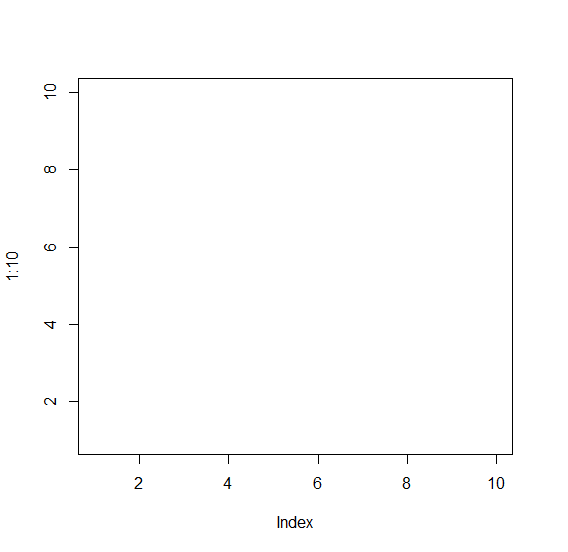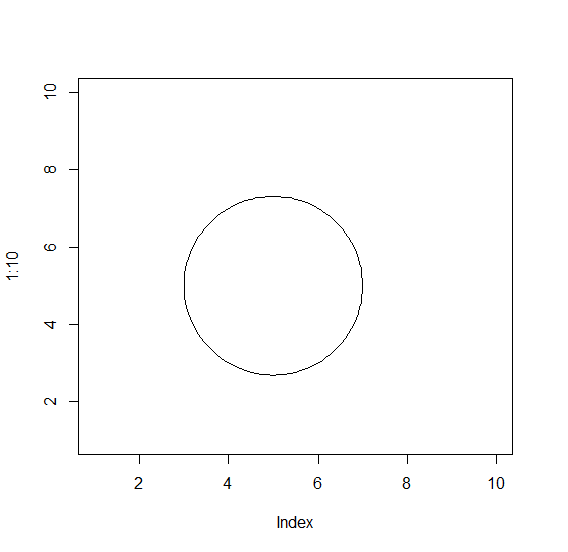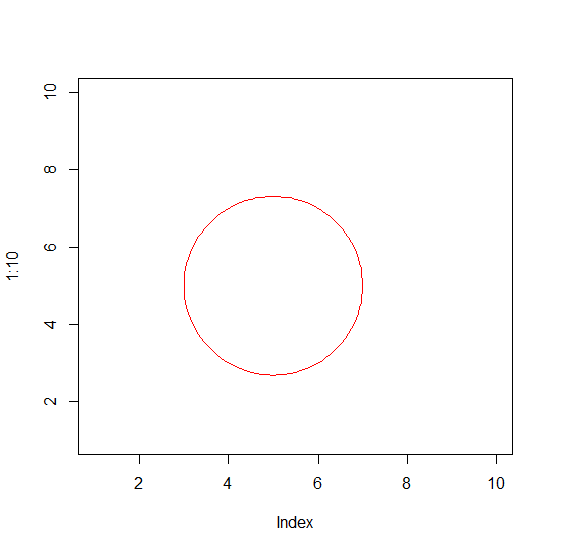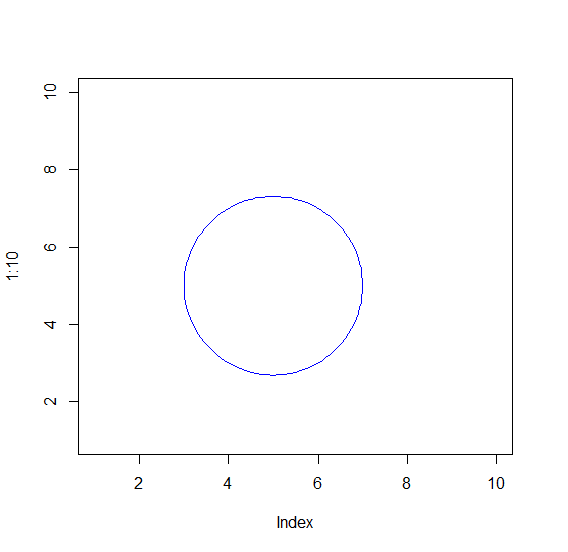# How to create a circle with different color border in R?

We can create a circle in R by using draw.circle function of plotrix package and default border color of the circle is black. If we want to change the border color of a circle then we can use border argument and pass the desired colors.

For Example, if we want to create a blue colored circle then, we can use the below mentioned command −

draw.circle(5,5,2,border="blue")

Check out the below Example to understand how it works.

## Example

To create a colored circle add the following code to the above snippet −

plot(1:10,type="n")

## Output

If you execute the above given snippet, it generates the following Output −To create a colored circle add the following code to the above snippet −

plot(1:10,type="n")
library(plotrix)
draw.circle(5,5,2)

## Output

If you execute all the above given snippets as a single program, it generates the following Output −To create colored circle add the following code to the above snippet −

plot(1:10,type="n")
library(plotrix)
draw.circle(5,5,2,border="red")

## Output

If you execute all the above given snippets as a single program, it generates the following Output −To create colored circle add the following code to the above snippet −

plot(1:10,type="n")
library(plotrix)
draw.circle(5,5,2,border="blue")

## Output

If you execute all the above given snippets as a single program, it generates the following Output −